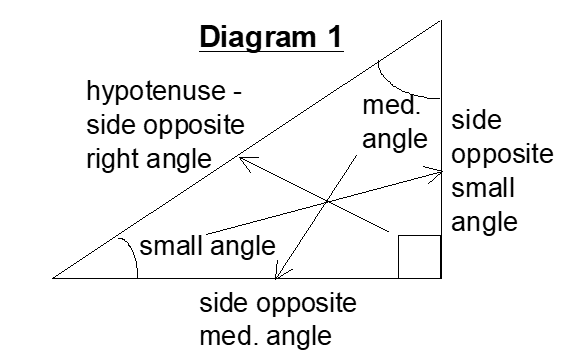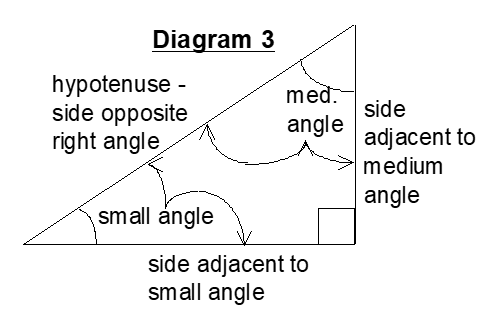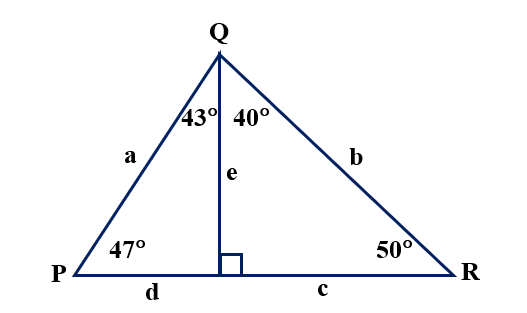# GEOM 2 | Lesson 4 | Try This! (Labeling Sides)## Labeling the Sides of Right Triangles

The common way to label the sides of right triangles is to name the two sides that form the right angle the legs, and the remaining side (also the longest side) the hypotenuse.Mathematicians have developed a way to tell the legs apart based on their position relative to one of the acute angles in the triangle.  The words they use to describe the legs are opposite and adjacent.

Every side is opposite (or across from) one of the angles of the triangle.  The smallest side is always opposite the smallest angle, the medium side is always opposite the  medium angle, and the longest side is always opposite the largest angle.  (See Diagram 1.)Since the longest side of the triangle will always be opposite the largest angle (the right angle), that side is named the hypotenuse.  (See Diagram 2.)The word adjacent means connected to the angle or next to the angle.  Each angle has two sides that are adjacent (touching or next to).  One of those sides is called the hypotenuse and the other side is called adjacent.  (See Diagram 3.)Use right triangle vocabulary to answer the following questions.1. How long is the hypotenuse of the right triangle on the left?
2. How long is the hypotenuse of the right triangle on the right?
3. How long is the side opposite the 43° angle?
4. How long is the side adjacent to the 43° angle?
5. How long is the side opposite the 50° angle?
6. How long is the side adjacent to the 50° angle?
7. How long is the side opposite the 47° angle?
8. How long is the side adjacent to the  40° angle?

Check solutions here.